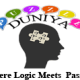Find the latest bookmaker offers available across all uk gambling sites www.bets.zone Read the reviews and compare sites to quickly discover the perfect account for you.
Home / Aptitude / Problems on Numbers – Solve with Intelligence

# Problems on Numbers – Solve with Intelligence

When you are playing with different numbers aptitude, there are very easy chance that your thought process is going through very clinical phases. You think every problem with defined logic and going to resolve with. But the immense satisfaction of solving them, is always hidden into the effort you put behind.Here are 7 interesting questions are placed with their answer options and proper explanation:

1. Find a positive number which when increased by 17 is equal to 60 times the reciprocal of the number.

A. 3
B. 7
C. 17
D. 24

3

### Explanation

Then, x + 17 =     60/x

x2 + 17x – 60 = 0

(x + 20)(x – 3) = 0

x = 3.

————————————————————————————————2. In a two-digit, if it is known that its unit’s digit exceeds its ten’s digit by 2 and that the product of the given number and the sum of its digits is equal to 144, then the number is:

A. 22
B. 24
C. 38
D. 44

24

### Explanation

Let the ten’s digit be x.

Then, unit’s digit = x + 2.

Number = 10x + (x + 2) = 11x + 2.

Sum of digits = x + (x + 2) = 2x + 2.

(11x + 2)(2x + 2) = 144

22×2 + 26x – 140 = 0

11×2 + 13x – 70 = 0

(x – 2)(11x + 35) = 0

x = 2.

Hence, required number = 11x + 2 = 24.

————————————————————————————————

3. A number consists of 3 digits whose sum is 10. The middle digit is equal to the sum of the other two and the number will be increased by 99 if its digits are reversed. The number is:

A. 135
B. 253
C. 368
D. 422

253

### Explanation

Let the middle digit be x.

Then, 2x = 10 or x = 5. So, the number is either 253 or 352.

Since the number increases on reversing the digits, so the hundred’s digits is smaller than the unit’s digit.

Hence, required number = 253.

————————————————————————————————

4. The product of two numbers is 9375 and the quotient, when the larger one is divided by the smaller, is 15. The sum of the numbers is:

A. 340
B. 390
C. 400
D. 455

400

### Explanation

Let the numbers be x and y.

Then, xy = 9375 and   x/y = 15.

xy/(x/y) = 9375/15

so, y2 = 625.

or, y = 25.

or, x = 15y = (15 x 25) = 375.Sum of the numbers = x + y = 375 + 25 = 400.

————————————————————————————————

5. The product of two numbers is 120 and the sum of their squares is 289. The sum of the number is:

A. 10
B. 23
C. 179
D. None of these

23

### Explanation

Let the numbers be x and y.

Then, xy = 120 and x2 + y2 = 289.

(x + y)2 = x2 + y2 + 2xy = 289 + (2 x 120) = 529

x + y = Sq. rt of529 = 23.

————————————————————————————————

6. What is the sum of two consecutive even numbers, the difference of whose squares is 84?

A. 30
B. 36
C. 42
D. 56

42

### Explanation

Let the numbers be x and x + 2.

Then, (x + 2)2 – x2 = 84

4x + 4 = 84

4x = 80

x = 20.

The required sum = x + (x + 2) = 2x + 2 = 42.

————————————————————————————————

7. The sum of two number is 25 and their difference is 13. Find their product.

A. 108
B. 114
C. 325
D. 387

114

### Explanation

Let the numbers be x and y.

Then, x + y = 25 and x – y = 13.

4xy = (x + y)2 – (x- y)2

= (25)2 – (13)2

= (625 – 169)

= 456

xy = 114.